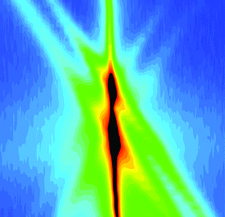issue contentsFOUNDATIONSADVANCES
ISSN: 2053-2733

March 2004 issueCover illustration: Diffuse scattering about the 002 reflection of an AlAs/GaAs superlattice grown on an oriented GaAs substrate. The in-plane and out-of plane components of the momentum transfer vector are oriented horizontally and vertically, respectively. The observed features are explained on the basis of the distorted-wave Born approximation. See Chernov et al. [Acta Cryst. (2003), A59, 551-559].

quantum crystallography

Acta Cryst. (2004). A60, 103
doi: 10.1107/S0108767304002788

Spectral momentum densities in matter determined by electron scattering

Electron momentum spectroscopy measurements and cumulant expansion many-body theoretical calculations are used to study the role of electron correlations in the ground electronic states of graphite and polycrystalline aluminium.

Electron localization functions obtained from X-ray constrained Hartree–Fock wavefunctions for mol­ecular crystals of ammonia, urea and alloxan

Images of a number of electron localization functions derived from X-ray constrained Hartree–Fock wavefunctions are presented for molecular crystals of ammonia, urea and alloxan.

research papers

Time-dependent Takagi–Taupin eikonal theory of X-ray diffraction in rapidly changing crystal structures

The Takagi–Taupin theory is synthesized with the ray–optical eikonal theory with time dependence explicitly included. Use of the dispersion surfaces in a unified space–time approach is demonstrated for diffraction under the conditions of crystal structure changes on the subpicosecond time scale. The theory is based upon microscopic electromagnetism and thus treats the spatial and temporal coordinates in the same way.

Ab initio structure solution by charge flipping

An amazingly simple structure solution algorithm is presented. It works ab initio on high-resolution data in the manner of Fourier recycling, in real space the sign of the charge density below a threshold is simply flipped.

A five-dimensional model of dodecagonal Ta–Te quasicrystals with fractal occupation domains

A five-dimensional model of dodecagonal Ta–Te quasicrystals is given. The two crystalline approximant structures can be derived from the model.

The crystallographic fast Fourier transform. IV. FFT-asymmetric units in the reciprocal space

Shapes are discussed of FFT-asymmetric units in the reciprocal space, compatible with our crystallographic FFT algorithms. An algorithm is presented for automatic generation of such shapes and points in the reciprocal space are characterized according to their isotropy subgroups. It is shown how both forward and inverse Fourier transforms can be calculated in-place.

Statistical approach to the phase problem

A statistically based formulation of the phase problem as one of constrained global optimization is proposed and tested.

Hexagonal and trigonal sphere packings. II. Bivariant lattice complexes

All homogeneous sphere packings, interpenetrating sphere packings and interpenetrating sphere layers were derived which refer to the bivariant lattice complexes of the hexagonal crystal family. Examples of corresponding crystal structures are given.

Magnetically ordered quasicrystals: enumeration of spin groups and calculation of magnetic selection rules

It is shown how to enumerate spin point groups and spin-space-group types for quasicrystals and use them to calculate selection rules for neutron diffraction experiments. Two-dimensional octagonal quasicrystals are considered as an example.

Symmetry of magnetically ordered three-dimensional octagonal quasicrystals

All three-dimensional octagonal spin point groups and spin-space-group types are enumerated and used to calculate selection rules for neutron scattering experiments.

short communications

`Exact' formulation for π-polarization waves of dynamical X-ray diffraction

Exact and self-consistent formulae for treating π-polarization two-beam X-ray diffraction from single crystals are refined within the Laue formalism.

Integral lattices

Integral lattices (characterized by an integral metric tensor) are revealed by peaks in the frequency distribution of hexagonal and tetrahedral inorganic compounds and in the molecular form of a RNA quadruplex.
Follow Acta Cryst. A